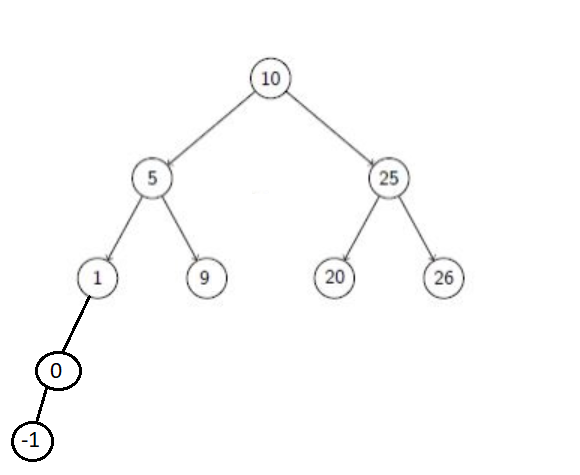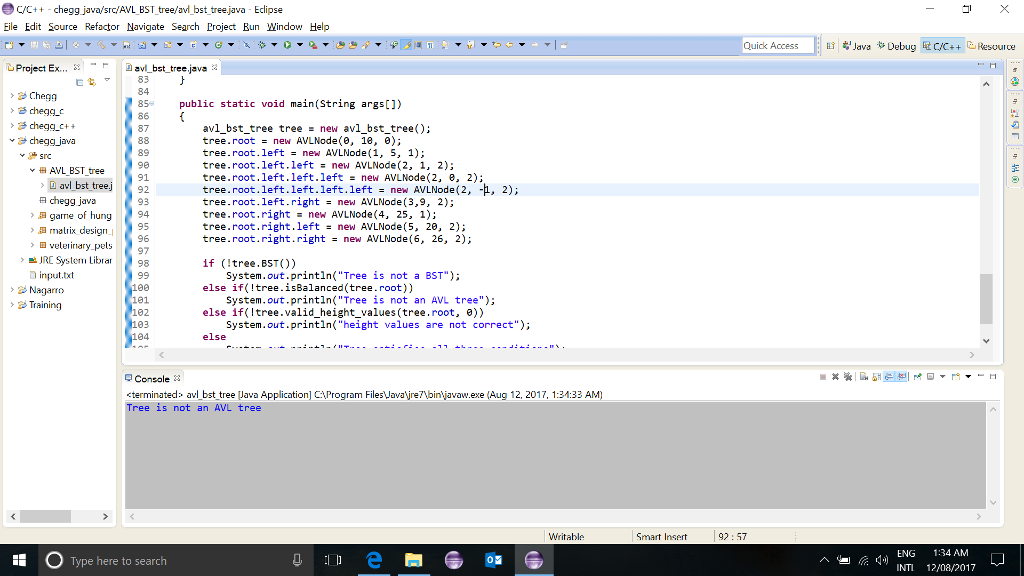# Question & Answer: Write code for an theta(n) worst-case algorithm that verifies that a tree is actually an AVL tree. You may assume the nodes of the tree look like: cl…..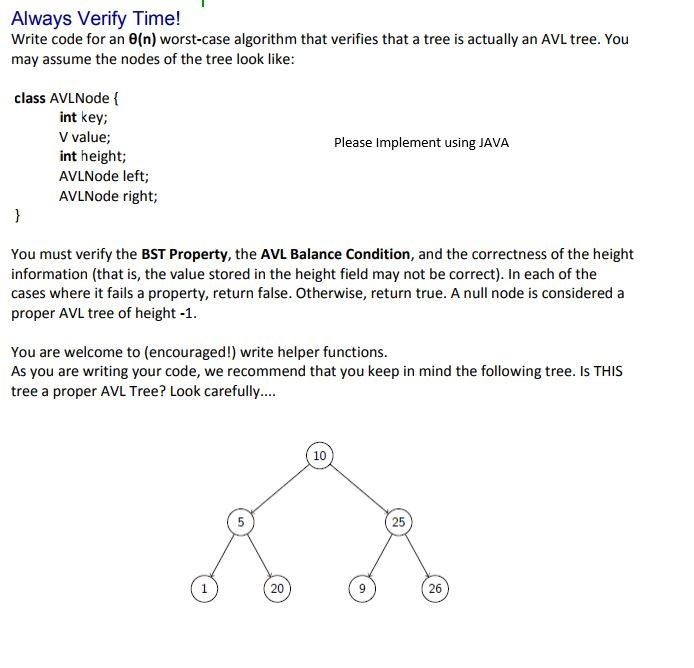Write code for an theta(n) worst-case algorithm that verifies that a tree is actually an AVL tree. You may assume the nodes of the tree look like: class AVLNode { int key: V value: int height: AVLNode left: AVLNode right: } Please Implement using JAVA You must verify the BST Property, the AVL Balance Condition, and the correctness of the height information (that is, the value stored in the height field may not be correct). In each of the cases where it fails a property, return false. Otherwise, return true. A null node is considered a proper AVL tree of height -1. You are welcome to (encouraged!) write helper functions. As you are writing your code, we recommend that you keep in mind the following tree. Is THIS tree a proper AVL Tree? Look carefully….

Don't use plagiarized sources. Get Your Custom Essay on
Question & Answer: Write code for an theta(n) worst-case algorithm that verifies that a tree is actually an AVL tree. You may assume the nodes of the tree look like: cl…..
GET AN ESSAY WRITTEN FOR YOU FROM AS LOW AS \$13/PAGE

Code and input/output is attached:

package AVL_BST_tree;

class AVLNode{
int key;
int value;
int height;
AVLNode left;
AVLNode right;

public AVLNode(int k, int val, int h){
this.key = k;
this.value = val;
this.height = h;
left = null;
right = null;
}
}

public class avl_bst_tree {
AVLNode root;
AVLNode prev;

int height(AVLNode node)
{
/* if tree is empty */
if(node == null)
return 0;
/*height = 1 + max(right height,left height) if tree is not empty*/
return 1 + Math.max(height(node.left), height(node.right));
}

// check if binary tree is height-balanced or not
boolean isBalanced(AVLNode root){
if(root==null){
return true;
}

// height of left and right subtree
int rh = height(root.right);
int lh = height(root.left);

//if balanced return true
if(Math.abs(rh-lh)<=1 && isBalanced(root.left) && isBalanced(root.right)){
return true;
}

return false;
}

//compare original height with written height(recursive)
boolean valid_height_values(AVLNode root, int h){
if(root==null){
return true;
}
else if(root.height != h){
return false;
}
else{
return (valid_height_values(root.left,h+1) && valid_height_values(root.right,h+1));
}
}

// check if tree is valid binary tree
boolean BST(){
prev = null;
return BST(root);
}

boolean BST(AVLNode root){
if(root!=null){
// check if left subtree is BST
if(!BST(root.left)){
return false;
}
// if current node is <= parent node
if(prev !=null && root.value<= prev.value){
return false;
}
prev = root;
return BST(root.right);
}
return true;
}

public static void main(String args[])
{
avl_bst_tree tree = new avl_bst_tree();
tree.root = new AVLNode(0, 10, 0);
tree.root.left = new AVLNode(1, 5, 1);
tree.root.left.left = new AVLNode(2, 1, 2);
tree.root.left.right = new AVLNode(3, 20, 2);
tree.root.right = new AVLNode(4, 25, 1);
tree.root.right.left = new AVLNode(5, 9, 2);
tree.root.right.right = new AVLNode(6, 26, 2);

if (!tree.BST())
System.out.println(“Tree is not a BST”);
else if(!tree.isBalanced(tree.root))
System.out.println(“Tree is not an AVL tree”);
else if(!tree.valid_height_values(tree.root, 0))
System.out.println(“height values are not correct”);
else
System.out.println(“Tree satisfies all three conditions”);
}

}

Sample input/output:

​1)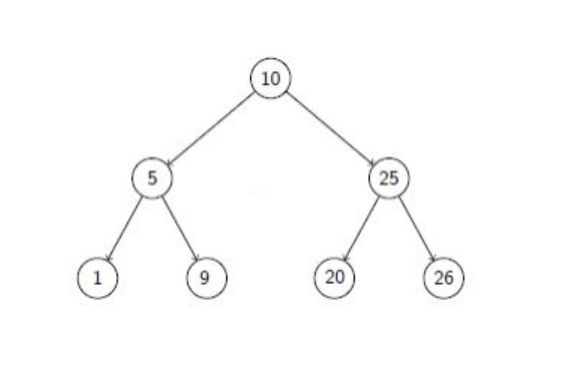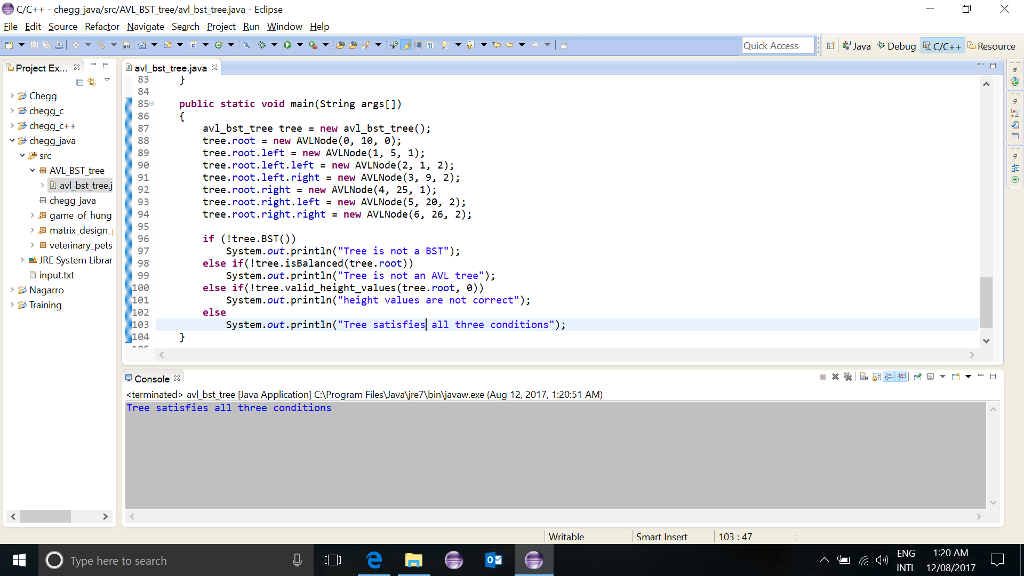2)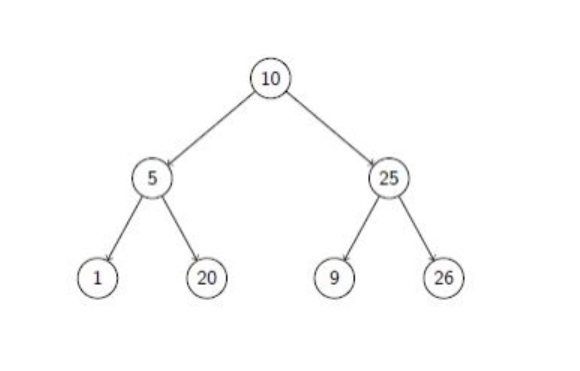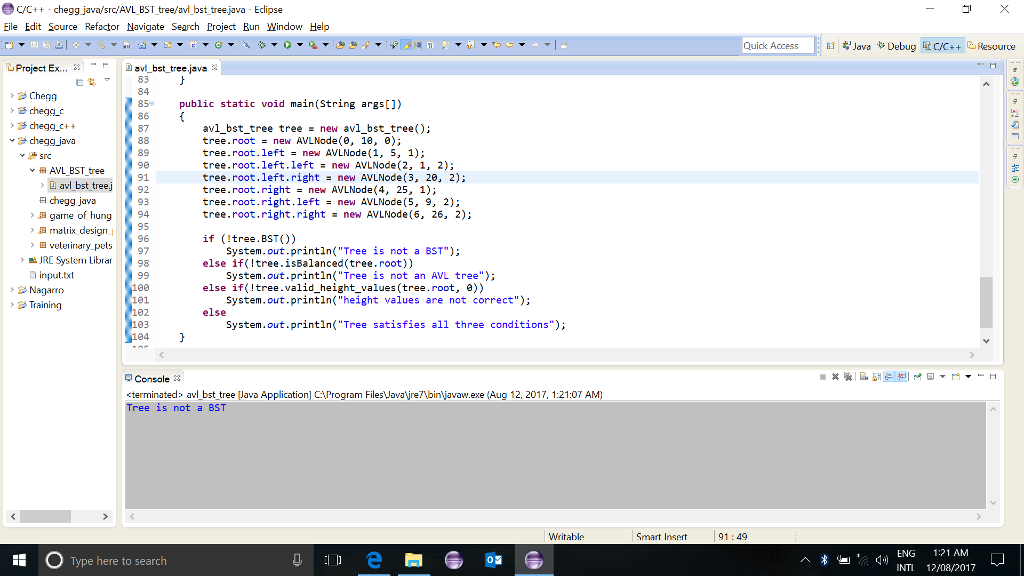3)